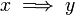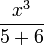# User talk:Emanuele

(Difference between revisions)
 Revision as of 22:19, 14 January 2010 (view source)Emanuele (Talk | contribs)← Older edit Revision as of 22:48, 14 January 2010 (view source)Emanuele (Talk | contribs) Newer edit → Line 3: Line 3: $x \implies y$ $x \implies y$ - $\frac{x^2}{5}$ + $\frac{x^3}{5+6}$

## Revision as of 22:48, 14 January 2010

testing math equations$x \implies y$$\frac{x^3}{5+6}$